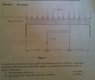# Deflection in a simply supported beam with a rigid arm underneath

cjh200
The attached question shows a simply supported beam with a UDL of 30kN/m and has a rigid arm underneath with a load. This question was a research one but unfortunately I have had no luck.

I thought that this could be solved by doing moments for the arm but am unsure on whether that would work for this question. If anyone can point me in the right direction would be much appreciated.

#### Attachments

•Q1.jpg
22.5 KB · Views: 474

Homework Helper
The question is testing to see if you know if the additional load acts at 0.5m or 1.0m.

that.kid
Have you drawn a free body diagram of the beam yet? That would be my starting point.

Firstly, resolve the UDL into a point force, acting equidistant between the points where the UDL is applied. force on the arm will create a moment where the arm is attached to the rigid beam, which is approximate to the force applied on the arm multiplied by the horizontal length of the arm. Once you have resolved the forces into a free body diagram, you can then draw a shear force diagram and a bending moment diagram.

That's about as far as I can get you right now off the top of my head. Conceptually, shear force and bending moment diagrams should help, as the maximum deflection should occur where the shear force is at its greatest (can someone check me on that please?). by doing a piecewise analysis of the shear force diagram you could easily find its horizontal distance (x), and being a uniformly distributed load, the shear force diagram will act linearly.

I'm guessing that that E=4*10^6 is some kind of young's modulus or something? Is there more to this question that is missing?

cjh200
Thanks for the fast response. The 4*10^-6 was E*I (youngs modulus*Inertia) and your response that.kid reconfirmed my idea of using moments to figure out the deflection. Once again thanks